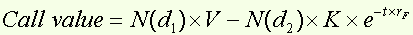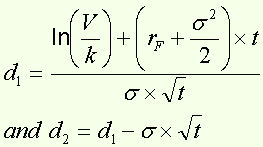Black-Scholes formula

Around the formula...

In a now famous article, Fisher Black and Myron Scholes (1972) presented a model for pricing European-style options that is now very widely used . For a call option, the Black & Scholes formula is as follows :with :where : V = current price of the underlying asset N(d) is a cumulative standard normal distribution (average 0, standard deviation 1) K = option's strike price  e = exponential function = 2,71828 rF = continual annual risk-free rate s = instantaneous standard deviation of the return on the underlying asset t = time remaining until maturity (in years) and ln = Naperian logarithm